login  home  contents  what's new  discussion  bug reports help  links  subscribe  changes  refresh  edit

References

## 2-d Example

Use LinearOperator (LOP)

fricas
(1) -> )lib CARTEN MONAL PROP LOP
CartesianTensor is now explicitly exposed in frame initial
CartesianTensor will be automatically loaded when needed from
/var/aw/var/LatexWiki/CARTEN.NRLIB/CARTEN
Monoidal is now explicitly exposed in frame initial
Monoidal will be automatically loaded when needed from
/var/aw/var/LatexWiki/MONAL.NRLIB/MONAL
Prop is now explicitly exposed in frame initial
Prop will be automatically loaded when needed from
/var/aw/var/LatexWiki/PROP.NRLIB/PROP
LinearOperator is now explicitly exposed in frame initial
LinearOperator will be automatically loaded when needed from
/var/aw/var/LatexWiki/LOP.NRLIB/LOP
L:=LOP(OVAR ['1,'2], EXPR INT)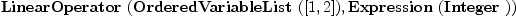(1)
Type: Type
fricas
-- basis
dx:=basisIn()$L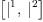(2) Type: List(LinearOperator(OrderedVariableList([1,2]),Expression(Integer))) fricas Dx:=basisOut()$L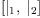(3)
Type: List(LinearOperator(OrderedVariableList([1,2]),Expression(Integer)))
fricas
-- summation
macro Σ(f,b,i) == reduce(+,[f*b.i for i in 1..#b])
Type: Void
fricas
-- identity
I:L:=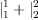(4)
Type: LinearOperator(OrderedVariableList([1,2]),Expression(Integer))
fricas
-- twist
X:L:=[2,1]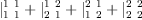(5)
Type: LinearOperator(OrderedVariableList([1,2]),Expression(Integer))
fricas
-- co-evaluation
Λ:L:=co(1)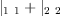(6)
Type: LinearOperator(OrderedVariableList([1,2]),Expression(Integer))
fricas
-- evaluation
V:L:=ev(1)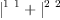(7)
Type: LinearOperator(OrderedVariableList([1,2]),Expression(Integer))

## Algebra

An n-dimensional algebra is represented by a (2,1)-tensor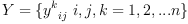viewed as a linear operator with two inputs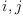and one output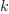. For example in 2 dimensions

fricas
Y:=Σ(Σ(Σ(script(y,[[i,j],[k]]),dx,i),dx,j),Dx,k)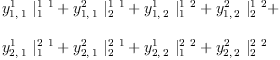(8)
Type: LinearOperator(OrderedVariableList([1,2]),Expression(Integer))

## Multiplication

Given two vectors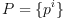and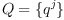fricas
P:=Σ(script(p,[[],[i]]),Dx,i)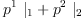(9)
Type: LinearOperator(OrderedVariableList([1,2]),Expression(Integer))
fricas
Q:=Σ(script(q,[[],[i]]),Dx,i)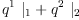(10)
Type: LinearOperator(OrderedVariableList([1,2]),Expression(Integer))

the tensor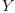operates on their tensor product to yield a vector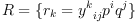fricas
R:=(P,Q)/Y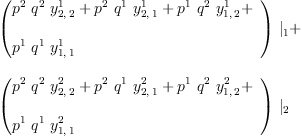(11)
Type: LinearOperator(OrderedVariableList([1,2]),Expression(Integer))

Pictorially:

  P Q
Y
R

or more explicitly

Pi Qj
\/
\
Rk


## Associator

An algebra is said to be associative if:

    Y    =    Y
Y       Y

i   j   k   i  j     k   i     j  k
\  |  /     \/     /     \     \/
\ | /       \    /       \    /
\|/    =    e  k    -    i  e
|           \/           \/
|            \           /
l             l         l


This requires that the following (3,1)-tensor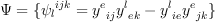(12)
(associator) is zero.
fricas
YY := (Y,I)/Y - (I,Y)/Y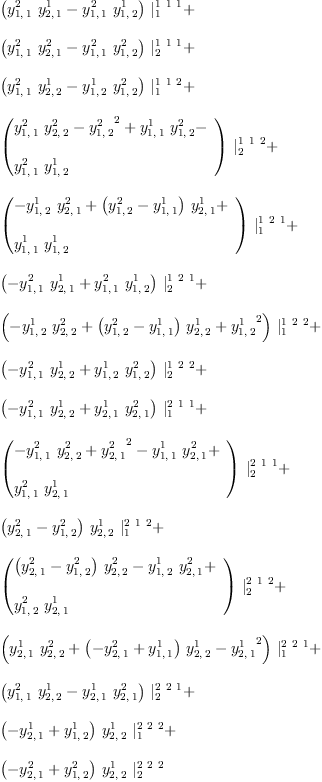(13)
Type: LinearOperator(OrderedVariableList([1,2]),Expression(Integer))

## Commutator

The algebrais commutative if:

    Y = Y

i   j     i  j     j  i
\ /   =   \/   -   \/
|         \       /
k          k     k


This requires that the following (2,1)-tensor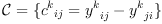(14)
(commutator) is zero.
fricas
YC:=Y-(X/Y)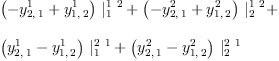(15)
Type: LinearOperator(OrderedVariableList([1,2]),Expression(Integer))

A basis for the ideal defined by the coefficients of the commutator is given by:

fricas
groebner(map(x+->x,ravel(YC))$LIST2(EXPR INT,POLY INT))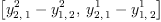(16) Type: List(Polynomial(Integer)) ## Anti-commutator The algebrais anti-commutative if:  Y = -Y i j i j j i \ / = \/ = \/ | \ / k k k  This requires that the following (2,1)-tensor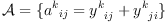(17) (anti-commutator) is zero. fricas YA:=Y+(X/Y)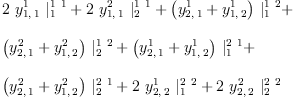(18) Type: LinearOperator(OrderedVariableList([1,2]),Expression(Integer)) A basis for the ideal defined by the coefficients of the commutator is given by: fricas groebner(map(x+->x,ravel(YA))$LIST2(EXPR INT,POLY INT))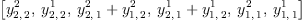(19)
Type: List(Polynomial(Integer))

## Jacobi

The Jacobi identity is:

              X
Y =  Y + Y
Y  Y     Y

i     j     k  i      j     k  i     j      k   i  j   k
\    |    /    \    /     /    \     \    /     \  \ /
\   |   /      \  /     /      \     \  /       \  0
\  |  /        \/     /        \     \/         \/ \
\ | /          \    /          \    /           \  \
\|/     =      e  k      -     i  e       -     e  j
|              \/              \/               \/
|               \              /                /
l                l            l                 l


An algebra satisfies the Jacobi identity if and only if the following (3,1)-tensor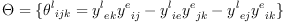(20)
is zero.

fricas
YX := YY - (I,X)/(Y,I)/Y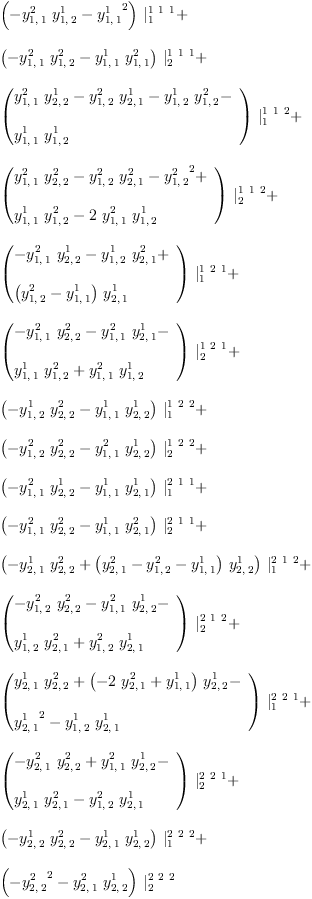(21)
Type: LinearOperator(OrderedVariableList([1,2]),Expression(Integer))

## Scalar Product

A scalar product is denoted by the (2,0)-tensor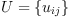fricas
U:=Σ(Σ(script(u,[[],[i,j]]),dx,i),dx,j)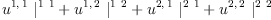(22)
Type: LinearOperator(OrderedVariableList([1,2]),Expression(Integer))

## Definition 1

We say that the scalar product is associative if the tensor equation holds:

    Y   =   Y
U     U


In other words, if the (3,0)-tensor:

    i  j  k   i  j  k   i  j  k
\ | /     \/  /     \  \/
\|/   =   \ /   -   \ /
0         0         0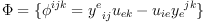(23)
(three-point function) is zero.

fricas
YU := (Y,I)/U - (I,Y)/U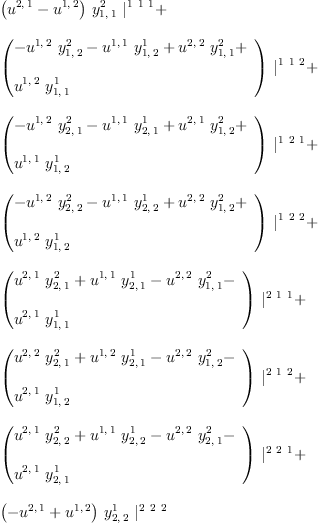(24)
Type: LinearOperator(OrderedVariableList([1,2]),Expression(Integer))

## Definition 2

An algebra with a non-degenerate associative scalar product is called pre-Frobenius.

We may consider the problem where multiplication Y is given, and look for all associative scalar products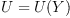or we may consider an scalar product U as given, and look for all algebras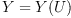such that the scalar product is associative.

This problem can be solved using linear algebra.

fricas
)expose MCALCFN
MultiVariableCalculusFunctions is now explicitly exposed in frame
initial
K := jacobian(ravel(YU),concat(map(variables,ravel(Y)))::List Symbol);
Type: Matrix(Expression(Integer))
fricas
yy := transpose matrix [concat(map(variables,ravel(Y)))::List Symbol];
Type: Matrix(Polynomial(Integer))
fricas
K::OutputForm * yy::OutputForm = 0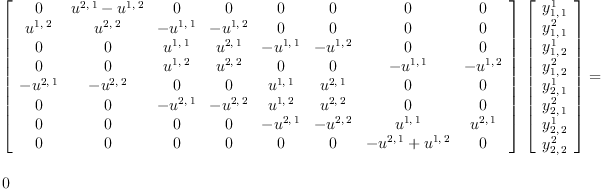(25)
Type: Equation(OutputForm?)

The matrix K transforms the coefficients of the tensorinto coefficients of the tensor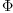. We are looking for coefficients of the tensor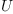such that K transforms the tensorinto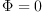for any.

A necessary condition for the equation to have a non-trivial solution is that the matrix K be degenerate.

## Theorem 1

All 2-dimensional pre-Frobenius algebras are symmetric.

Proof: Consider the determinant of the matrix K above.

fricas
Kd := factor(determinant(K)::DMP(concat map(variables,ravel(U)),FRAC INT))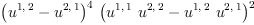(26)
Type: Factored(DistributedMultivariatePolynomial?([u[;1,1],u[;1,2],u[;2,1],u[;2,2]],Fraction(Integer)))

The scalar product must also be non-degenerate

fricas
Ud:DMP(concat map(variables,ravel(U)),FRAC INT) := determinant [[retract((Dx.i,Dx.j)/U) for j in 1..#Dx] for i in 1..#Dx]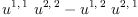(27)
Type: DistributedMultivariatePolynomial?([u[;1,1],u[;1,2],u[;2,1],u[;2,2]],Fraction(Integer))

therefore U must be symmetric.

fricas
nthFactor(Kd,1)
There are no exposed library operations named nthFactor but there
are 3 unexposed operations with that name. Use HyperDoc Browse or
issue
)display op nthFactor
Cannot find a definition or applicable library operation named
nthFactor with argument type(s)
Factored(DistributedMultivariatePolynomial([u[;1,1],u[;1,2],u[;2,1],u[;2,2]],Fraction(Integer)))
PositiveInteger
Perhaps you should use "@" to indicate the required return type,
or "$" to specify which version of the function you need. ## Theorem 2 All 2-dimensional algebras with associative scalar product are commutative. Proof: The basis of the null space of the symmetric K matrix are all symmetric fricas YUS := (I,Y)/US - (Y,I)/US There are 15 exposed and 15 unexposed library operations named / having 2 argument(s) but none was determined to be applicable. Use HyperDoc Browse, or issue )display op / to learn more about the available operations. Perhaps package-calling the operation or using coercions on the arguments will allow you to apply the operation. Cannot find a definition or applicable library operation named / with argument type(s) Tuple(LinearOperator(OrderedVariableList([1,2]),Expression(Integer))) Variable(US) Perhaps you should use "@" to indicate the required return type, or "$" to specify which version of the function you need.

This defines a 4-parameter family of 2-d pre-Frobenius algebras

fricas
test(eval(YUS,SS)=0*YUS)
There are 10 exposed and 6 unexposed library operations named eval
having 2 argument(s) but none was determined to be applicable.
Use HyperDoc Browse, or issue
)display op eval
package-calling the operation or using coercions on the arguments
will allow you to apply the operation.
Cannot find a definition or applicable library operation named eval
with argument type(s)
Variable(YUS)
Variable(SS)
Perhaps you should use "@" to indicate the required return type,
or "\$" to specify which version of the function you need.

Alternatively we may consider

fricas
J := jacobian(ravel(YU),concat(map(variables,ravel(U)))::List Symbol);
Type: Matrix(Expression(Integer))
fricas
uu := transpose matrix [concat(map(variables,ravel(U)))::List Symbol];
Type: Matrix(Polynomial(Integer))
fricas
J::OutputForm * uu::OutputForm = 0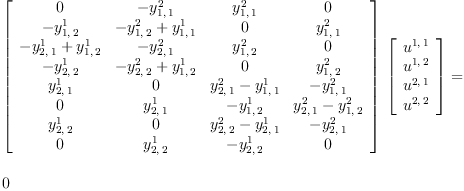(28)
Type: Equation(OutputForm?)

The matrix J transforms the coefficients of the tensorinto coefficients of the tensor. We are looking for coefficients of the tensorsuch that J transforms the tensorintofor any.

A necessary condition for the equation to have a non-trivial solution is that all 70 of the 4x4 sub-matrices of J are degenerate. To this end we can form the polynomial ideal of the determinants of these sub-matrices.

fricas
JP:=ideal concat concat concat
[[[[ determinant(
matrix([row(J,i1),row(J,i2),row(J,i3),row(J,i4)]))::FRAC POLY INT
for i4 in (i3+1)..maxRowIndex(J) ]
for i3 in (i2+1)..(maxRowIndex(J)-1) ]
for i2 in (i1+1)..(maxRowIndex(J)-2) ]
for i1 in minRowIndex(J)..(maxRowIndex(J)-3) ];
Type: PolynomialIdeal?(Fraction(Integer),IndexedExponents?(Symbol),Symbol,Polynomial(Fraction(Integer)))
fricas
#generators(%)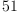(29)
Type: PositiveInteger?

## Theorem 3

If a 2-d algebra is associative, commutative, anti-commutative or if it satisfies the Jacobi identity then it is a pre-Frobenius algebra.

Proof

Consider the ideals of the associator, commutator, anti-commutator and Jacobi identity

fricas
YYI:=ideal(ravel(YY)::List FRAC POLY INT);
Type: PolynomialIdeal?(Fraction(Integer),IndexedExponents?(Symbol),Symbol,Polynomial(Fraction(Integer)))
fricas
in?(JP,YYI)  -- associative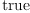(30)
Type: Boolean
fricas
YCI:=ideal(ravel(YC)::List FRAC POLY INT);
Type: PolynomialIdeal?(Fraction(Integer),IndexedExponents?(Symbol),Symbol,Polynomial(Fraction(Integer)))
fricas
in?(JP,YCI)  -- commutative(31)
Type: Boolean
fricas
YAI:=ideal(ravel(YA)::List FRAC POLY INT);
Type: PolynomialIdeal?(Fraction(Integer),IndexedExponents?(Symbol),Symbol,Polynomial(Fraction(Integer)))
fricas
in?(JP,YAI)  -- anti-commutative(32)
Type: Boolean
fricas
YXI:=ideal(ravel(YX)::List FRAC POLY INT);
Type: PolynomialIdeal?(Fraction(Integer),IndexedExponents?(Symbol),Symbol,Polynomial(Fraction(Integer)))
fricas
in?(JP,YXI) -- Jacobi identity(33)
Type: Boolean

## Y-forms

Three traces of two graftings of an algebra gives six (2,0)-forms.

Left snail and right snail:

  LS                    RS

Y /\                    /\ Y
Y  )                  (  Y
\/                    \/

i  j                        j  i
\/                          \/
\    /\              /\    /
e  f  \            /  f  e
\/    \          /    \/
\    /          \    /
f  /            \  f
\/              \/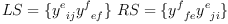(34)

fricas
LS:=
( Y Λ  )/ _
(  Y I )/ _
V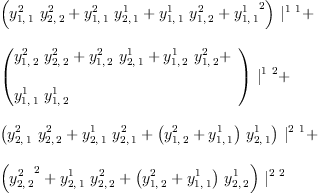(35)
Type: LinearOperator(OrderedVariableList([1,2]),Expression(Integer))
fricas
RS:=
(  Λ Y )/ _
( I Y  )/ _
V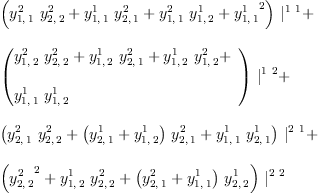(36)
Type: LinearOperator(OrderedVariableList([1,2]),Expression(Integer))
fricas
test(LS=RS)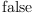(37)
Type: Boolean

Left and right deer:

   RD                 LD

\ /\/              \/\ /
Y /\              /\ Y
Y  )            (  Y
\/              \/

i            j    i            j
\    /\    /      \    /\    /
\  f  \  /        \  /  f  /
\/    \/          \/    \/
\    /\          /\    /
e  /  \        /  \  e
\/    \      /    \/
\    /      \    /
f  /        \  f
\/          \/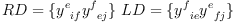(38)
Left and right deer forms are identical but different from snails.
fricas
RD:=
(  I Λ I  ) / _
(   Y X   ) / _
(    Y I  ) / _
V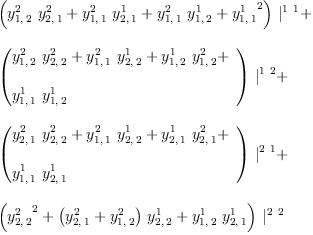(39)
Type: LinearOperator(OrderedVariableList([1,2]),Expression(Integer))
fricas
LD:=
(  I Λ I  ) / _
(   X Y   ) / _
(  I Y    ) / _
V(40)
Type: LinearOperator(OrderedVariableList([1,2]),Expression(Integer))
fricas
test(LD=RD)(41)
Type: Boolean
fricas
test(RD=RS)(42)
Type: Boolean
fricas
test(RD=LS)(43)
Type: Boolean

Left and right turtles:

  RT                   LT

/\ / /               \ \ /\
(  Y /                 \ Y  )
\  Y                   Y  /
\/                     \/

i     j      i     j
/\    /     /        \     \    /\
/  f  /     /          \     \  f  \
/    \/     /            \     \/    \
\     \    /              \    /     /
\     e  /                \  e     /
\     \/                  \/     /
\    /                    \    /
\  f                      f  /
\/                        \/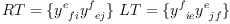(44)

fricas
RT:=
(  Λ I I ) / _
( I Y I  ) / _
(  I Y   ) / _
V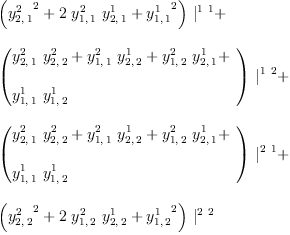(45)
Type: LinearOperator(OrderedVariableList([1,2]),Expression(Integer))
fricas
LT:=
( I I Λ  ) / _
(  I Y I ) / _
(   Y I  ) / _
V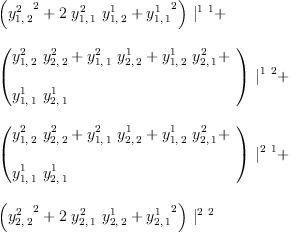(46)
Type: LinearOperator(OrderedVariableList([1,2]),Expression(Integer))
fricas
test(LT=RT)(47)
Type: Boolean

The turles are symmetric

fricas
test(RT = X/RT)(48)
Type: Boolean
fricas
test(LT = X/LT)(49)
Type: Boolean

Five of the six forms are independent.

fricas
test(RT=RS)(50)
Type: Boolean
fricas
test(RT=LS)(51)
Type: Boolean
fricas
test(RT=RD)(52)
Type: Boolean
fricas
test(LT=RS)(53)
Type: Boolean
fricas
test(LT=LS)(54)
Type: Boolean
fricas
test(LT=RD)(55)
Type: Boolean

Associativity implies right turtle equals right snail and left turtle equals left snail.

fricas
in?(ideal(ravel(RT-RS)::List FRAC POLY INT),YYI)(56)
Type: Boolean
fricas
in?(ideal(ravel(LT-LS)::List FRAC POLY INT),YYI)(57)
Type: Boolean

If the Jacobi identity holds then both snails are zero

fricas
in?(ideal(ravel(RS)::List FRAC POLY INT),YXI)(58)
Type: Boolean
fricas
in?(ideal(ravel(LS)::List FRAC POLY INT),YXI)(59)
Type: Boolean

and right turtle and deer have opposite signs

fricas
in?(ideal(ravel(RT+RD)::List FRAC POLY INT),YXI)(60)
Type: Boolean

 Subject:   Be Bold !! ( 14 subscribers )